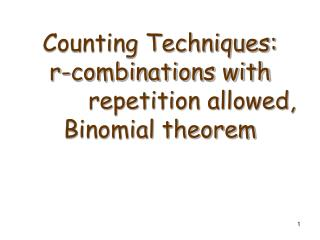# Counting Techniques: r-combinations with repetition allowed, Binomial theorem - PowerPoint PPT PresentationDownload PresentationCounting Techniques: r-combinations with repetition allowed, Binomial theorem

Download Presentation## Counting Techniques: r-combinations with repetition allowed, Binomial theorem

- - - - - - - - - - - - - - - - - - - - - - - - - - - E N D - - - - - - - - - - - - - - - - - - - - - - - - - - -
##### Presentation Transcript

1. Counting Techniques:r-combinations with repetition allowed,Binomial theorem

2. Number of iterations of a nested loop(First Situation) • Consider the following nested loop: for i:=1 to n for j:=1 to i-1 for k:=1 to j-1 [ Statements] next k next j next i • Question: How many times the statements in the innermost loop will be executed? • Solution: Each iteration corresponds to a triple of integers (i, j, k) where i > j > k . The set of all this kind of triples corresponds to all 3-combinations of {1, …, n} . Thus, the total number of iterations is C(n,3).

3. Number of iterations of a nested loop(Second Situation) • Consider the following nested loop: for i:=1 to n for j:=1 to i for k:=1 to j [ Statements] next k next j next i • Question: How many times the statements in the innermost loop will be executed? • Solution: Each iteration corresponds to a triple of integers (i, j, k) where i ≥ j ≥ k . Examples: (5, 3, 2), (4, 4, 3), (2, 1, 1), (3, 3, 3) . How to count the number of this kind of triples?

4. Number of iterations of a nested loop(Second Situation) Each triple corresponds to a string of crosses and vertical bars. • Numbers 1, 2, …, n are considered as categories; • n-1 vertical bars separate the categories; • 3 crosses indicate how many items from each category are chosen. Examples when n=5:

5. Number of iterations of a nested loop(Second Situation) • Each triple corresponds to a string of n-1 vertical bars and 3 crosses. The length of any string is (n-1)+3 = n+2 . The number of distinct positions for the 3 crosses in a string is C(n+2, 3) . Thus, the number of distinct triples is C(n+2, 3) . • Generalizing, if the number of nested loops is r then the number of iterations is C(n-1+r, r) .

6. r-combinations with repetition allowed • Definition: r-combinations with repetition allowed from a set X of n elements is an unordered selection of r elements taken from X with repetition allowed. • The number of r-combinations with repetition allowed from a set of n elements is C(n-1+r, r) .

7. Which formula to use? • We discussed four different ways of choosing r elements from n . • The summary of formulas used in the four situations:

8. Useful formulas for special cases • C(n,n-r) = C(n,r) . Proof: • C(n,n)=1 • C(n,n-1) = C(n,1) = n • C(n,n-2) = C(n,2) = n(n-1) / 2

9. Binomial Theorem Theorem: For any real numbers a and b and nonnegative integer n, Proof: By distributive law, (a+b)n can be expanded into products of n letters where each letter is a or b :

10. Binomial Theorem Proof(cont.): For each k=0,1,…, n, the product an-kbkoccurs as a term in the sum the same number of times as the number of ways to choose k positions for b’s. But this number is C(n,k). Thus, the coefficient of an-kbk is C(n,k) .■ Examples: (a+b)2 = a2+C(2,1)∙ab+b2 = a2+2∙ab+b2 (a+b)3 = a3+C(3,1)∙a2b+C(3,2)∙ab2+b3 = a3+3∙a2b+3∙ab2+b3

11. Using the Binomial Theorem • Question: Which number is larger: (1.2)4,000 or 800 ? • Solution: By the binomial theorem, (1.2)4,000 = (1+.2)4,000 =14000 + C(4000, 1)∙13999∙.2 + other positive terms =1 + 4000∙1∙.2 + other positive terms =1 + 800 + other positive terms > 800

12. Using the Binomial Theorem